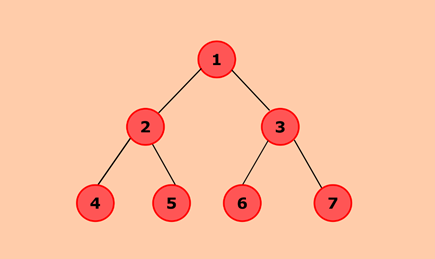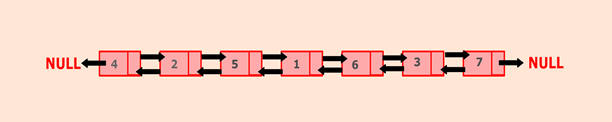Q:

# Java program to convert a given binary tree to doubly linked list

belongs to collection: Java Doubly Linked List Programs

In this program, we need to convert the given binary tree to corresponding doubly liked list.

The binary tree is a tree data structure in which each node has at most two children node.

This can be achieved by traversing the tree in the in-order manner that is, left the child -> root ->right node. Traverse left sub-tree and convert it into the doubly linked list by adding nodes to the end of the list. In this way, leftmost node will become head of the list. Then, convert the right sub-tree into the doubly linked list.### Algorithm

• Define a Node class which represents a node in the binary tree. It will have three properties: data left, and right where the left and right represent two children of a node.
• Root will represent the root of the binary tree. Head and tail node represent the head and tail of the doubly linked list.
• BinaryTreeToDLL() will convert the given binary tree to corresponding doubly linked list.
• Perform inorder traversal of the binary tree by converting the left subtree first.
• If the list is empty, both head and tail will point to a node.
• If the list is not empty, the node will be inserted at the end of the list. Here, pointer left, and right will represent the previous and next pointer of the doubly linked list.
• Now, recursively call BinaryTreeToDLL() to convert the right subtree.

a. display() will show all the nodes present in the list.

• Define a new node 'current' that will point to the head.
• Print current.data till current points to null.
• Current will point to the next node in the list in each iteration.

### Program:

``````public class BinaryTreeToDLL {
//Represent a node of the binary tree
public static class Node{
int data;
Node left;
Node right;

public Node(int data) {
this.data = data;
this.left = null;
this.right = null;
}
}

//Represent the root of the binary tree

public Node root;

//convertbtToDLL() will convert the given binary tree to corresponding doubly linked list
public void convertbtToDLL(Node node) {
//Checks whether node is null
if(node == null)
return;

//Convert left subtree to doubly linked list
convertbtToDLL(node.left);

//Both head and tail will point to node
}
//Otherwise, add node to the end of the list
else {
//node will be added after tail such that tail's right will point to node
tail.right = node;
//node's left will point to tail
node.left = tail;
//node will become new tail
tail = node;
}

//Convert right subtree to doubly linked list
convertbtToDLL(node.right);
}

//display() will print out the nodes of the list
public void display() {
//Node current will point to head
System.out.println("List is empty");
return;
}
System.out.println("Nodes of generated doubly linked list: ");
while(current != null) {
//Prints each node by incrementing the pointer.

System.out.print(current.data + " ");
current = current.right;
}
System.out.println();
}

public static void main(String[] args) {

BinaryTreeToDLL bt = new BinaryTreeToDLL();
//Add nodes to the binary tree
bt.root = new Node(1);
bt.root.left = new Node(2);
bt.root.right = new Node(3);
bt.root.left.left = new Node(4);
bt.root.left.right = new Node(5);
bt.root.right.left = new Node(6);
bt.root.right.right = new Node(7);

//Converts the given binary tree to doubly linked list
bt.convertbtToDLL(bt.root);

//Displays the nodes present in the list
bt.display();
}
}    ``````

Output:

```Nodes of generated doubly linked list:
4 2 5 1 6 3 7 ```